# Bernoulli's Equation

Also found in: Dictionary.
The following article is from The Great Soviet Encyclopedia (1979). It might be outdated or ideologically biased.

## Bernoulli’s Equation

a basic equation of hydrodynamics that connects (for a steady flow) the velocity of a flowing fluid v, its pressure ρ, and the height h of the location of a small volume of fluid above a reference plane. Bernoulli’s equation was derived by D. Bernoulli in 1738 for a small stream of ideal, incompressible fluid of constant density ρ which is under the influence only of gravitational force. In this case, Bernoulli’s equation takes the form

v2/2 + p/p + gh = constant

where g is the acceleration of gravity. If this equation is multiplied by ρ, then the first term will represent the kinetic energy of a unit volume of fluid, and the other two terms will represent the potential energy, one part of which is determined by the force of gravity (the last term of the equation) and the other part by the pressure ρ. Bernoulli’s equation in such a form expresses the law of conservation of energy. If one form of energy—for example, kinetic—is increasing along the fluid stream, then the potential energy decreases by the same amount. Therefore, for example, upon reduction (narrowing) of the stream flowing in a tube, when the flow velocity increases (since in the same time period the same quantity of fluid passes through the narrowed cross section as through the larger cross section), the pressure in the tube decreases correspondingly. (The principle of operation of the venturi tube is based on this phenomenon.)

A number of important consequences follow from Bernoulli’s equation. For example, in the discharge of a fluid from an open vessel under the influence of gravity (see Figure 1), it follows from Bernoulli’s equation that v 2/2g = h, or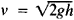—that is, the velocity of the fluid at the outlet aperture is the same as that of fluid particles falling freely from the height h.

If a uniform flow of fluid with velocity v0 and pressure p0 encounters an obstacle along its path (see Figure 2), then a backwater—a retardation of the flow—arises directly in front of the obstacle; in the center of the backwater region, at the critical point, the flow velocity is equal to zero. From Bernoulli’s equation, it follows that the pressure at the critical point is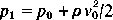. The pressure increment at this point, equal to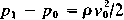, is called the dynamic pressure or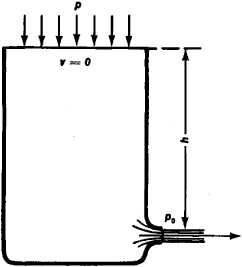Figure 1. Discharge from an open vessel

the velocity head. In a stream of real fluid, its mechanical energy is not conserved along the flow, but is used up in doing frictional work and dissipated in the form of thermal energy; consequently, in the application of Bernoulli’s equation to a real fluid, it is necessary to take into account the losses caused by drag.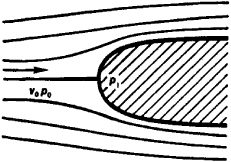Figure 2. Flow around an obstacle

Bernoulli’s equation is of great importance in hydraulics and technical hydrodynamics: it is used in pipeline and pump calculations, in the solution of problems involving filtration, and so on. Bernoulli’s equation for a medium with variable density p, together with the mass constancy equation and the equation of state, form the basis of gas dynamics.

### REFERENCES

Fabrikant, N. Ia. Aerodinamika, parts 1–2. Leningrad, 1949–64.
Uginchus, A. A. Gidravlika, gidravlicheskie mashiny i osnovy sel’skokhoziaistvennogo vodosnabzheniia. Kiev-Moscow, 1957. Chapter 5.
References in periodicals archive ?
The unsteady Bernoulli's equation for any points on the moving boundaries of the liquid domain in the Lagrangian form is given:
The purpose of this work was to investigate "how does an exit pipe affect the calculation of drainage time of water from a tank using Bernoulli's equation?" in an attempt to study the situational limitations of Bernoulli's equation.
Based on the Bernoulli's equation in cross sections 1-1 and 2-2 (Fig.
Using the hydrostatic pressure equation at arbitrary height, z, and the Bernoulli's equation for velocity, the mass flow rate from compartment i to compartment j through an opening is given by
Bernoulli's equation (Equation 5) states the change in pressure, kinetic energy, and potential energy is equal to the mechanical energy ([delta][W.sub.s]) added to the system minus the viscous losses ([L.sub.v]).
Subsonic airflow satisfies conservation of mass as well as Bernoulli's equation so the ratio of test area to nozzle area can be calculated given the pressure developed by the fan and the desired test velocity.
This principle defines the conservation of momentum, which leads to Bernoulli's equation when viscous forces are neglected, steady flow and constant density are assumed.
Solutions were obtained using (1) a three-dimensional solution of the Navier-Stokes equation using computational fluid dynamics and (2) Bernoulli's equation and a momentum balance in one dimension.
It is governed by the simple gravity form of Bernoulli's equation of energy,
The authors said current runner systems are troublesome because gating design calculations make intensive use of Bernoulli's equation and the law of continuity.
If the burner doesn't have a dedicated gas or oil meter, Bernoulli's equation provides a way to approximate burner turndown when firing gas.
Each component's influence on the total filter resistance was then formulated through the use of Forchheimer-extended Darcy's Law, Bernoulli's equation, and the equation of continuity.

Site: Follow: Share:
Open / Close